# 2D Tilemap Collision

## Introduction

Collision detection and response is a black art within game development. It's required in huge variety of games, but is sufficiently maths-y and hidden to make it a challenging subject for newcomers. If you don't want to, or are not able to use a form of collision detection/response built into your platform it can be very hard to start.

Most existing tutorials focus on advanced problems, but it seems useful to put down some of what I've learnt about the basics. Hopefully it might make starting out a little less bewildering.

## Terminology

When I talk about collision detection I mean determining whether two (or more) objects are overlapping. It is, for example, checking if a ball is sitting near the grass, vs sitting on the grass.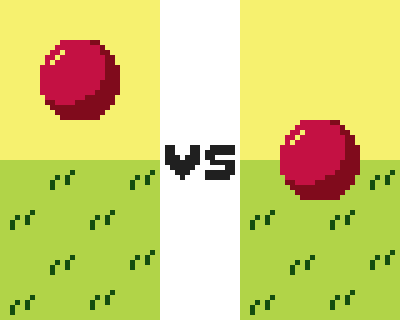Collision response follows on from collision detection, and is typically thought of as a part of 'collision' when discussing games. Collision response is what happens to an object in your game after collision has been detected, for example, preventing a ball from travelling through a wall.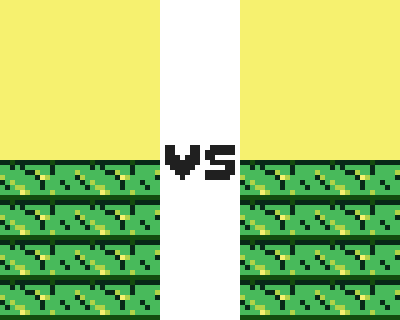It is typically collision response we are after, but collision detection is crucial in figuring out if there has been a collision to respond to.

## Detecting Rectangle Collision

When we consider collision and response we are abstracting behaviour we see in real physical objects. We simplify the behaviour so that we can program it.

The most useful simplification of collision for 2D games is collision between two non-rotating rectangles. This gets called other things: axis-aligned bounding boxes, aabb intersection etc. but they all amount to the same thing.

If you have round objects, or complicated shapes (like people!) you might think that simplifying them to rectangles is too much. Sometimes it is, but usually it's not.

We can consider a rectangle to be determined by four values, a left, a right, a top and a bottom. In most 2D game coordinates left and right are 'x' values, left value lower than the right; top and bottom are 'y' values, top value numerically lower than the bottom.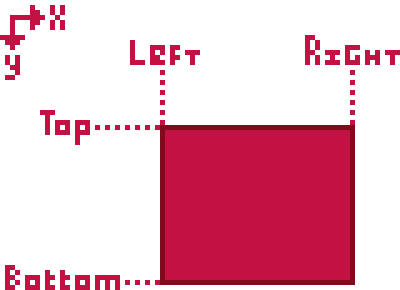To detect collision between two rectangles we can check if the two rectangles 'x' values (left+right) overlap. We can also check if the rectangles 'y' values (top+bottom) overlap. If an overlap occurs between both 'x' and 'y' values the two rectangles are overlapping, otherwise they are not.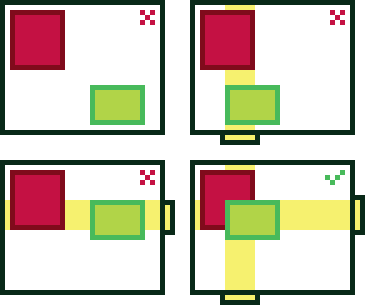Given two rectangles, A and B. The 'x' values overlap if A's left value is less than B's right AND A's right value is greater than B's left.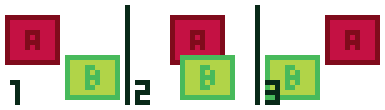1. A's left is less than B's right, but A's right is LESS than B's left. No overlap.
2. A's left is less than B's right. A's right is greater than B's left. Overlap!
3. A's right is greater than B's right but A's left is also GREATER than B's right. No overlap.

The code for checking for an 'x' overlap might look something like this

``````x_overlaps = (a.left < b.right) && (a.right > b.left)
``````

Similarly the 'y' values overlap if A's top value is less than B's bottom AND A's bottom is greater than B's top.

``````y_overlaps = (a.top < b.bottom) && (a.bottom > b.top)
``````

Then we combine those terms to check for collision.

``````x_overlaps = (a.left < b.right) && (a.right > b.left)
y_overlaps = (a.top < b.bottom) && (a.bottom > b.top)
collision = x_overlaps && y_overlaps
``````

We can then use this on any pair of rectangles to detect collision.

## Detecting Tile Collision

In a 2D game a tilemap is typically a grid of even-sized rectangular (usually square) tiles. Each tile might contain information about it's appearance, but also an indication of whether the tile is a wall, open space, or something else.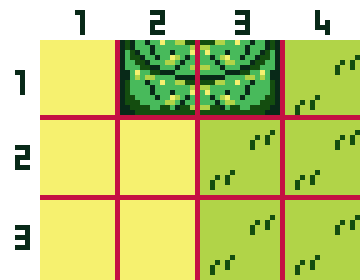Given we have a method of detecting collision between two rectangles, detecting collision between an object and a tilemap can be as simple as comparing a rectangle representing the object and every single wall tile rectangle.

We iterate over every tile in the tilemap, if the tile is a wall we compare the objects rectangle with the tiles. If any collisions occur between a wall tile and the objects rectangle, the object is colliding with the tilemap.

``````any_collision = false
for(i=0; i<tilemap.width; i++)
{
for(j=0; j<tilemap.height; j++)
{
tile t = tilemap.tile_at(i, j)
if(t.is_wall)
{
x_overlaps = (object.left < t.right) && (object.right > t.left)
y_overlaps = (object.top < t.bottom) && (object.bottom > t.top)
collision = x_overlaps && y_overlaps
if(collision)
{
any_collision = true
}
}
}
}
``````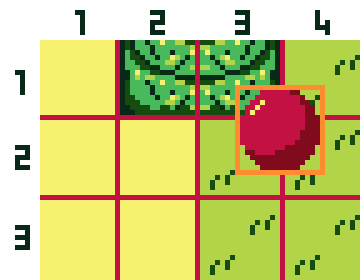In this example the first tile (1,1) is skipped because it is not a wall. The second tile (2,1) is checked, but does not overlap. The third tile (3,1) is checked and does overlap, so the object is colliding. All the rest of the tiles are quietly skipped because they are not walls.

## Optimising?

The above solution works great for a small tilemap but as tilemap size increases it becomes increasingly less viable. With a 16x16 tilemap only 256 checks are required per object, in a 128x128 tilemap 16384 are required per object. If performance becomes a concern it is possible to use the implicit positioning of tiles to greatly reduce the number of tiles that need to be checked.

If you calculate the 'x' tile position at the left and right value of the object rectangle, and the 'y' tile position at the top and bottom of the object rectangle. You can use these to only check the tiles that definitely overlap the object rectangle. This also means you can eliminate the rectangle overlap check.

``````int left_tile = object.left / tilemap.tile_width
int right_tile = object.right / tilemap.tile_width
int top_tile = object.top / tilemap.tile_height
int bottom_tile = object.bottom / tilemap.tile_height

if(left_tile < 0) left_tile = 0
if(right_tile > tilemap.width) right_tile = tilemap.width
if(top_tile < 0) top_tile = 0
if(bottom_tile > tilemap.height) bottom_tile = tilemap.height

any_collision = false
for(i=left_tile; i<=right_tile; i++)
{
for(j=top_tile; j<=bottom_tile; j++)
{
tile t = tilemap.tile_at(i, j)
if(t.is_wall)
{
any_collision = true
}
}
}
``````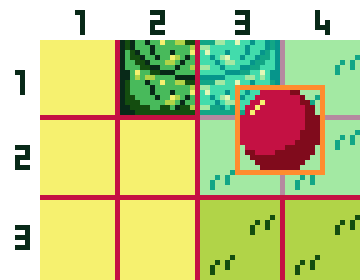The loops only iterate over four tiles (3,1) (4,1) (3,2) and (4,2). Of these (3,1) is a wall, so a collision occured.

## Collision Response

All the above only really covers half the picture. It is one thing to be able to determine if an object is colliding with a wall. It is another thing to take steps so that an object is prevented from colliding with a wall. As often as not it's the latter that we are really after.

Unlike collision detection, collision response is quite strongly tied into the way that an object is being moved, and the nature of the object it is colliding with. This makes it a little difficult to advise for all situations. Instead of attempting to do so, I will focus on a single scenario.This game is top-down controlled, think zelda-like. Each frame the character is given a speed based on joystick input, and her position is moved by that speed. I would like her movement to be halted by collision with a wall. I want it to feel as smooth and natural as possible.

## A Naive Attempt

The simplest thing we can attempt to prevent the character ending a frame overlapping a wall is to check her rectangle for collision against the tilemap, and to revert to her old position if a collision occured.

``````speed = joystick_speed()
old_position = girl.position
girl.position = girl.position + speed
collided = tilemap_collision(tilemap, girl.rectangle)
if(collided)
{
girl.position = old_position
}
``````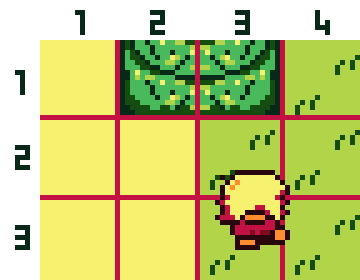This has some problems though, most problematically when the player is moving in both 'x' and 'y' coordinates. Instead of halting in the collision direction, and continuing to 'slide' along the wall in the other direction, all movement ceases. It feels like the character is stuck on the wall.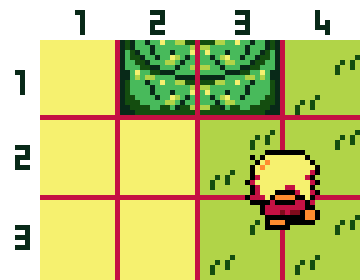## Wall Sliding

It is possible to resolve the 'sticky' wall issue by getting a mechanics book and diving into normals + impulses, but that gets technical and difficult fast. The cheap, effective alternative is to simply perform the 'x' move and the 'y' move as seperate steps.

The reason this is viable is because the tilemaps grid means we are only really concerned with smoothness of action along the axis. If we were to add diagonal tiles, and deal the collision detection for that, we would have to rethink this solution too.

So, we move the 'x' value, and then revert if the new position is colliding. We then move the 'y' value, and then revert that change if new position is colliding. It is fairly arbitrary whether to do 'x' or 'y' first, although in games in which the axis are treated asymmetrically you may improve the feel by picking one over the other. Consider experimenting.

The code might look something like this:

``````speed = joystick_speed()
old_position = girl.position
girl.position.x = girl.position.x + speed.x
collided = tilemap_collision(tilemap, girl.rectangle)
if(collided)
{
girl.position = old_position
}
old_position = girl.position
girl.position.y = girl.position.y + speed.y
collided = tilemap_collision(tilemap, girl.rectangle)
if(collided)
{
girl.position = old_position
}
``````

Now when a character is pushing diagonally against a wall the component of the movement which is blocked is discarded, and the component of the movement which is free still occurs.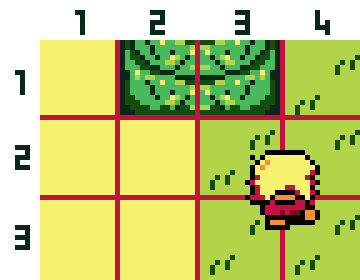## Sub-steps

A remaing problem is that this approach does not work well for objects moving at high speeds. Anything faster than 1 pixel per second can appear problematic.

This is because as we make and undo the provisional movement every single frame the character can 'float' over the surface it is meant to collide with. For example if it was 3 pixels from a wall, moving at 5 pixels per second the character would never actually close the distance to the wall; it would move 2 pixels into the wall, detect a collision, then move all the way back.

Another problem is that an object moving faster than the tile size might 'phase' past a wall entirely. This happens when the object moves beyond the wall within a single frame.

The simplest fix to both problems is to perform many smaller moves within a single frame, so that the relative speed for our collision code is much lower. If the character is unlikely to be travelling faster than 5 pixels a second, maybe we perform 5 incremental steps, instead of one 5 pixel move.

``````num_steps = 5
step_speed = joystick_speed() / num_steps
for(i=0; i<num_steps; i++)
{
... DO A MOVEMENT STEP USING step_speed INSTEAD OF joystick_speed() ...
}
``````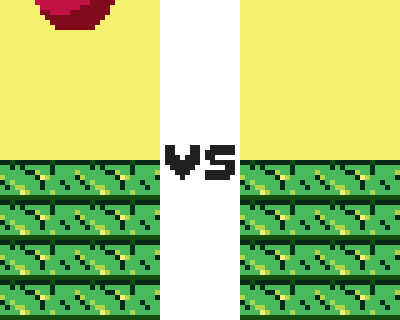The number of steps should be tuned to your particular game, though as long as your performance remains good it is fine to use a large number.

## Wrapping Up

Okay, hopefully that's enough for a useful starter guide. There's lots of further topics and fiddling that could be covered, but this should be enough to sort out collision for a simple tile-based game. If you have any questions or thoughts on how I might improve this guide please email jonathan@ignika.com or tweet at me @whitingjp

## Who Wrote This?

This guide was written by Jonathan Whiting. Thanks go to dock, for prompting its creation.